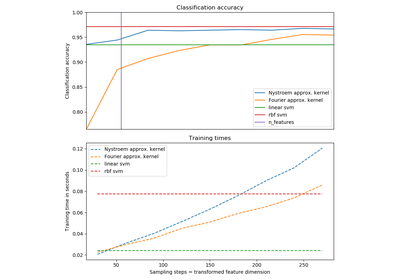# `sklearn.kernel_approximation`.Nystroem¶

class `sklearn.kernel_approximation.``Nystroem`(kernel=’rbf’, gamma=None, coef0=None, degree=None, kernel_params=None, n_components=100, random_state=None)[source]

Approximate a kernel map using a subset of the training data.

Constructs an approximate feature map for an arbitrary kernel using a subset of the data as basis.

Read more in the User Guide.

Parameters: kernel : string or callable, default=”rbf” Kernel map to be approximated. A callable should accept two arguments and the keyword arguments passed to this object as kernel_params, and should return a floating point number. n_components : int Number of features to construct. How many data points will be used to construct the mapping. gamma : float, default=None Gamma parameter for the RBF, laplacian, polynomial, exponential chi2 and sigmoid kernels. Interpretation of the default value is left to the kernel; see the documentation for sklearn.metrics.pairwise. Ignored by other kernels. degree : float, default=None Degree of the polynomial kernel. Ignored by other kernels. coef0 : float, default=None Zero coefficient for polynomial and sigmoid kernels. Ignored by other kernels. kernel_params : mapping of string to any, optional Additional parameters (keyword arguments) for kernel function passed as callable object. random_state : int, RandomState instance or None, optional (default=None) If int, random_state is the seed used by the random number generator; If RandomState instance, random_state is the random number generator; If None, the random number generator is the RandomState instance used by np.random. components_ : array, shape (n_components, n_features) Subset of training points used to construct the feature map. component_indices_ : array, shape (n_components) Indices of `components_` in the training set. normalization_ : array, shape (n_components, n_components) Normalization matrix needed for embedding. Square root of the kernel matrix on `components_`.

`RBFSampler`
An approximation to the RBF kernel using random Fourier features.
`sklearn.metrics.pairwise.kernel_metrics`
List of built-in kernels.

References

• Williams, C.K.I. and Seeger, M. “Using the Nystroem method to speed up kernel machines”, Advances in neural information processing systems 2001
• T. Yang, Y. Li, M. Mahdavi, R. Jin and Z. Zhou “Nystroem Method vs Random Fourier Features: A Theoretical and Empirical Comparison”, Advances in Neural Information Processing Systems 2012

Methods

 `fit`(X[, y]) Fit estimator to data. `fit_transform`(X[, y]) Fit to data, then transform it. `get_params`([deep]) Get parameters for this estimator. `set_params`(**params) Set the parameters of this estimator. `transform`(X) Apply feature map to X.
`__init__`(kernel=’rbf’, gamma=None, coef0=None, degree=None, kernel_params=None, n_components=100, random_state=None)[source]
`fit`(X, y=None)[source]

Fit estimator to data.

Samples a subset of training points, computes kernel on these and computes normalization matrix.

Parameters: X : array-like, shape=(n_samples, n_feature) Training data.
`fit_transform`(X, y=None, **fit_params)[source]

Fit to data, then transform it.

Fits transformer to X and y with optional parameters fit_params and returns a transformed version of X.

Parameters: X : numpy array of shape [n_samples, n_features] Training set. y : numpy array of shape [n_samples] Target values. X_new : numpy array of shape [n_samples, n_features_new] Transformed array.
`get_params`(deep=True)[source]

Get parameters for this estimator.

Parameters: deep : boolean, optional If True, will return the parameters for this estimator and contained subobjects that are estimators. params : mapping of string to any Parameter names mapped to their values.
`set_params`(**params)[source]

Set the parameters of this estimator.

The method works on simple estimators as well as on nested objects (such as pipelines). The latter have parameters of the form `<component>__<parameter>` so that it’s possible to update each component of a nested object.

Returns: self :
`transform`(X)[source]

Apply feature map to X.

Computes an approximate feature map using the kernel between some training points and X.

Parameters: X : array-like, shape=(n_samples, n_features) Data to transform. X_transformed : array, shape=(n_samples, n_components) Transformed data.

## Examples using `sklearn.kernel_approximation.Nystroem`¶Explicit feature map approximation for RBF kernels